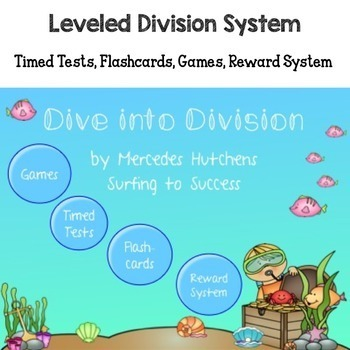Multiplication and Division Fact Fluency Bundle3rd - 4th
Subjects
Standards
Resource Type
Formats Included
• Zip
Pages
200+
\$19.25
Bundle
List Price:
\$27.50
You Save:
\$8.25
\$19.25
Bundle
List Price:
\$27.50
You Save:
\$8.25

Products in this Bundle (7)

showing 1-5 of 7 products

Description

Want your students to have fun while memorizing their basic multiplication and division facts? This bundle contains everything you need to help your students develop fact fluency in multiplication and division. Students work through step by step levels to multiply and divide fluently. Games, posters, word problems and fact family practice included!

Our students need a strong understanding of basic multiplication and division to be successful in math. This bundle contains a system you can set up in your classroom that can lead to students focusing on step by step goals. This system can lead to focused practice time with fun games. Once you introduce the games, students can play them as early finishers. Start with the multiplication system. Students that master the multiplication facts can move on to the division system. This system naturally differentiates and allows students to move at their own pace.

This bundle contains several resources. Check out all the previews!
Total Pages
200+
Included
Teaching Duration
1 Year
Report this Resource to TpT
Reported resources will be reviewed by our team. Report this resource to let us know if this resource violates TpT’s content guidelines.

Standards

to see state-specific standards (only available in the US).
Find all factor pairs for a whole number in the range 1-100. Recognize that a whole number is a multiple of each of its factors. Determine whether a given whole number in the range 1-100 is a multiple of a given one-digit number. Determine whether a given whole number in the range 1-100 is prime or composite.
Interpret a multiplication equation as a comparison, e.g., interpret 35 = 5 × 7 as a statement that 35 is 5 times as many as 7 and 7 times as many as 5. Represent verbal statements of multiplicative comparisons as multiplication equations.
Fluently multiply and divide within 100, using strategies such as the relationship between multiplication and division (e.g., knowing that 8 × 5 = 40, one knows 40 ÷ 5 = 8) or properties of operations. By the end of Grade 3, know from memory all products of two one-digit numbers.
Understand division as an unknown-factor problem. For example, find 32 ÷ 8 by finding the number that makes 32 when multiplied by 8.
Apply properties of operations as strategies to multiply and divide. Examples: If 6 × 4 = 24 is known, then 4 × 6 = 24 is also known. (Commutative property of multiplication.) 3 × 5 × 2 can be found by 3 × 5 = 15, then 15 × 2 = 30, or by 5 × 2 = 10, then 3 × 10 = 30. (Associative property of multiplication.) Knowing that 8 × 5 = 40 and 8 × 2 = 16, one can find 8 × 7 as 8 × (5 + 2) = (8 × 5) + (8 × 2) = 40 + 16 = 56. (Distributive property.)